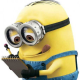## Converting a Float to a Char ArraySurprisingly, this question pops up often. While the technique used here is not really a conversion, it does allow access to the individual byte values of a float variable by constructing a union. A union is a data structure that can have several formats. In this example we make a union out of a float and 4-byte char array.

Reducing a float into it’s component bytes is useful, for example when limited to transmitting values one byte at a time (with no loss of precision).

Further information on unions can be found here.

Information on the float format and conversion can be found here.

A basic float-to-ascii (ftoa) function can be found here.

Arduino example program:

```union FloatChar {
float f;
char c[sizeof(float)];
};

FloatChar fc;

void setup(void) {
float f;

Serial.begin(9600);

f = 2.45;
fc.f = f;
for (int i=0; i<sizeof(float); i++)
Serial.println(fc.c[i]);

}

void loop(void) { }
```